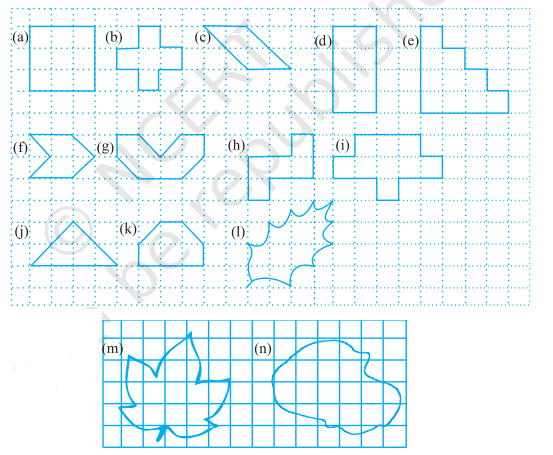# NCERT Class 6 Mathematics Tenth Chapter Mensuration Exercise 10.2 Solution

## EXERCISE 10.2

(1) Find the areas of the following figures by counting squareIn case you are missed :- Previous Chapter Exercise Solution

Ans: (a) 9 square units

(b) 5 square units

(c) 4 square units

(d) 8 square units

(e) 10 square units

(f) 4 square units

(g) 6 square units

(h) 5 square units

(i) 9 square units

(j) 4 square units

(k) 5 square units

(l) 8 square units

(m) 14 square units

(n) 18 square units

In case you are missed :- Next Chapter Exercise Solution

Updated: April 14, 2023 — 3:13 pm